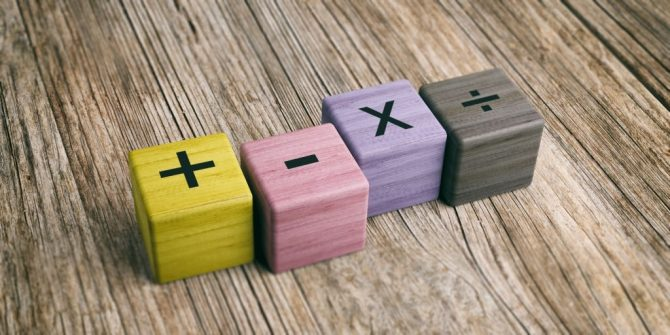5th grade mathematics often seems daunting due to the high level of complex thought that it requires.  No more straightforward facts from flash cards.  This year, students will use the foundation that they already have as they pick up and practice pre-algebra skills that will be used in middle school.  No doubt it can be overwhelming, but consistent practice will help students to master each concept on its own before applying them to more complex scenarios.

# Decimals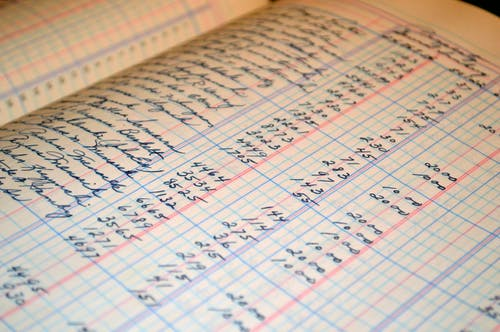## Equivalent Decimals

While this may seem like an easy concept, adding zeros to the end of a decimal can be confusing for some.  It is an essential skill for doing any operation with decimals, however, so students should understand equivalencies with added zeroes.

## Writing Decimals in Words and in Expanded Notation

Just as students learned expanded notation and place value in whole numbers, they must also master this skill in reading decimals.  Breaking down each part of the decimal into its place value allows them to write the number in words with expanded notation.

## Place Values

Learning how to properly identify numbers both before and after the decimal is an essential skill.  Students must learn the tenths, hundredths, and thousandths places.

## Comparing Decimals (Thousandths) & Ordering Decimals

Using a place value table can help 5th graders see the relationship between two decimals.  Often they will need to add a zero to the end to properly evaluate the two numbers and compare based on place value and then properly order them.

## Adding and Subtracting 3 Decimals

Learning how to line up numbers by the decimal is the first step in mastering these operations.  Filling in blanks with zeros will help when manipulating the numbers from right to left with addition or subtraction.

## Rounding Decimals

5th graders must first master place value before they can begin to round, as the rounding takes place based on the place value rounded.  Then they can use the rule of 5 to determine if it is rounded up or down.

## Multiplying by Powers of 10

A quick and easy way to multiply by powers of ten is accomplished by moving the decimal to the right equal to the number of zeroes there are in the number.  So, for example, if there are 3 zeros, you move the decimal three places to the right when multiplying.

# Fractions## Proper and Improper Fractions

When the numerator (number on top) is less than the denominator (number on bottom), the fraction is proper.  If the numerator is larger than the denominator, the fraction is improper.

## Add, Subtract with Unlike Denominators

Recognizing unlike denominators and factoring to find the lowest common denominator (LCD) can be difficult to master.  This requires not only multiplication skills, but also logic.  Once the fractions have a common denominator, they can be added or subtracted.

## Adding Mixed Numbers (Like & Unlike Denominators)

This process requires two parts:  adding the whole number and then adding the fraction.  If the fractions do not have an LCD, one must be calculated in order to complete the problem.

## Multiplying Fractions by Fractions & Whole Numbers

Multiplying fractions is very simple – you multiply the numerators by the numerators, and the denominators by the denominators.  There does not need to be a common denominator.  When multiplying by whole numbers, you change the whole number to a fraction by adding a 1 as the denominator before proceeding to multiply the numerators and then the denominators.

## Dividing Unit Fractions by Whole Numbers

Making a model fraction will help to visualize the division of the fraction into parts.  Further dividing the parts by the dividend will help you to determine the quotient.

## Dividing Whole Numbers by Unit Fractions

Making a model of the wholes and then dividing each by the unit fraction will help you to determine your answer.   Another way is to flip the unit fraction and multiply.

## Dividing Fractions by Fractions

To divide a fraction by another fraction, you simply multiply it by its reciprocal.  The second fraction in the number sentence is flipped (reversed) and then you straight multiply across the numerators and the denominators.

## Equivalent Fraction Patterns

Equivalent fractions have the same value or represent equal parts of a whole.  For example, ¼ is the same as 2/8.  You can obtain equivalent fractions by either multiplying or dividing both the numerator and the denominator by the same number.

## Mixed Numbers

When you combine a whole number and a proper fraction, you have a mixed number.  You can turn improper fractions into a mixed number by dividing the numerator by the denominator to get a whole number and using what’s left as a remainder.

# Geometry & Shapes## Rotational Symmetry

If a figure can be rotated about one point less than a full turn and still look the same as it did pre-rotation, then it has rotational symmetry.  However many times it can be turned is considered its “order of rotational symmetry.”

## Classifying Triangles

There are several different types of triangles depending on the equivalency of their sides and the size of their angles.  Different types include acute, obtuse, equilateral, isosceles, right, and scalene.

## Lines of Symmetry

A line of symmetry is the imaginary line drawn that will create mirror images if the object is folded on the line.  If they don’t match exactly, there isn’t a line of symmetry.

## Reflection, Rotation, & Translation

These are the three basic transformations that shapes can go through.  Reflection flips a shape to create a mirror image across a line.  Rotation turns a figure on a fixed point in a clockwise or counterclockwise rotation.  Translation moves or slides an image up, down, left, or right.

# Linear Functions## Function Tables

A table that displays the inputs and corresponding outputs of a function is a function table.  A function – or particular rule – details how the numbers are related

## Generating Patterns & Graphing Relationships

When you have an ordered pair – an x and a y coordinate – you can graph the results. Often input tables will help you to achieve a set of ordered pairs.

## Input/Output Tables

When the table is complete, you must determine the function or relationship between each pair of numbers.  This takes some thought and logical processing to accomplish to determine a rule.

# Mixed Operations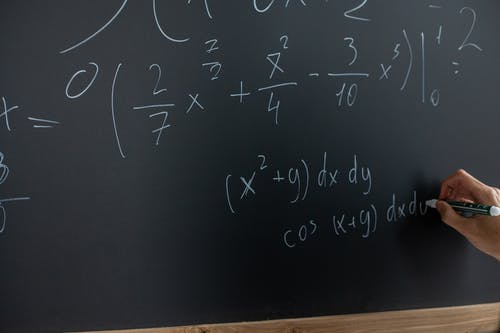## Order of Operations

Using the acronym PEMDAS can help 5th graders to remember the order in which mathematical operations must be performed in an equation.  Parentheses – Exponents – Multiplication & Division (from left to right) – Addition & Subtraction (from left to right).

## Input/Output Tables

When the table is complete, you must determine the function or relationship between each pair of numbers.  This takes some thought and logical processing to accomplish to determine a rule.

# Multiplication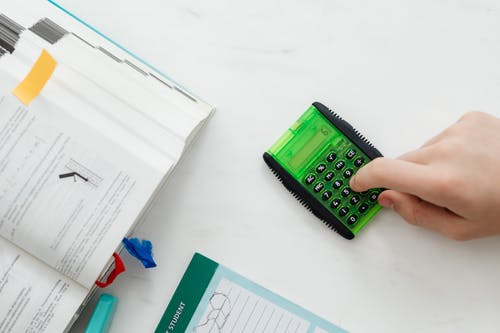## Multiply Numbers up to 3 Digits by 3-Digit Numbers

Lining up the numbers appropriately is the key to multiplying more than one number by another.  Then you follow a diagonal pattern, multiplying and adding any remainders to be added after the next diagonal multiplication, from right to left. Then you must move to the next number to the left and repeat the process, adding a zero as the placeholder in your answer.  Once you have completed this with all numbers, you add the second set together for your answer.

## Multiply Numbers up to 3 Digits by 2-Digit Numbers

Lining up the numbers appropriately is the key to multiplying more than one number by another.  Then you follow a diagonal pattern, multiplying and adding any remainders to be added after the next diagonal multiplication, from right to left.  Then you must move to the next number to the left and repeat the process, adding a zero as the placeholder in your answer.  Once you have completed this with all numbers, you add the second set together for your answer.

# Temperature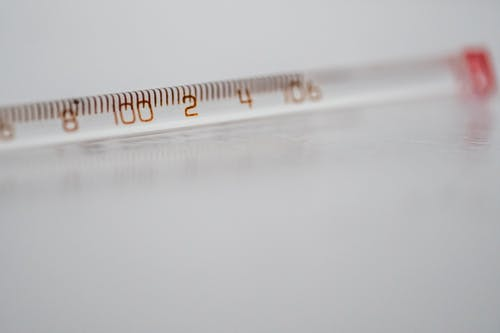## Reasonable Temperature

When considering base temperatures in Celcius and Fahrenheit for the human body, boiling point, and freezing temp, reasonable guesses can be made to determine an appropriate temperature for certain items or locations.

# Units of Measurement## Compare and Convert Metric Units of Weight

Being able to convert grams to larger or smaller units is as simple as multiplying or dividing by a power of ten, depending on place value.  Understanding the meaning of certain Latin prefixes like kilo- meaning 1,000 will make this process simpler.

## Compare and Convert Metric Units of Volume

Being able to convert liters to larger or smaller units is as simple as multiplying or dividing by a power of ten, depending on place value.  Understanding the meaning of certain Latin prefixes like milli- meaning 1/1,000 will make this process simpler.

# Variables## Variable Expressions

A variable is a letter that stands for an unknown quantity.  An expression means a group of numbers, symbols, and variables that represent another number.  Often these are translated into groups of words that all mean the same operation; for example, sum, more than, increased by, plus, and together all indicate addition.  Learning these words will make it easier for 5th graders to identify the operation indicated within a word problem.

5th grade can be an auspicious undertaking at first, but with practice and learning key words step by step, anyone can thrive in mathematics.  Taking time to practice each element can help these students to achieve success in any math classroom.